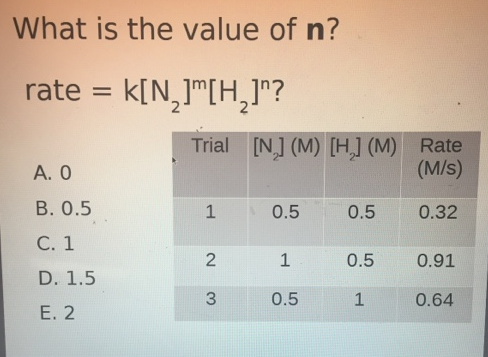# Problem: What is the value of n? rate = k[N2]m [H2]n? a. 0 b. 0.5 c. 1 d. 1.5 e. 2

###### FREE Expert Solution
86% (360 ratings)###### Problem Details

What is the value of n?

rate = k[N2]m [H2]n

a. 0

b. 0.5

c. 1

d. 1.5

e. 2